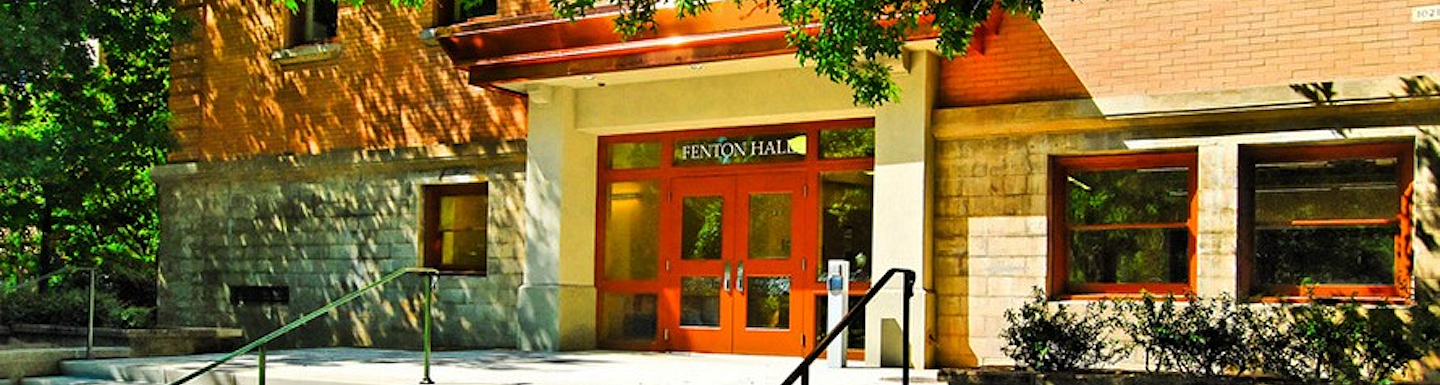# Courses

Undergraduate courses in the Department of Mathematics divide roughly into classical mathematical areas as follows. However, note that such a division is subjective because most areas of mathematics have interesting and deep relationships with many other areas. Students planning on graduate work are encouraged to take courses in several fields to get some feeling for the breadth of mathematics.

Analysis
The branch of mathematics dealing with calculus and its generalizations is called analysis. Courses in this area include advanced calculus, Introduction to Analysis (Math 316-317), Functions of a Complex Variable (Math 411-412), Differential Equations (Math 320 and 420), Fourier series (Math 421-422), and Numerical Analysis (Math 351-352).

Algebra
The study of sets with operations generally falls under the heading of algebra. Our courses in this area include Math 341, Math 342 and Math 441 in linear algebra; two sequences of abstract algebra (Math 391-392 and Math 444-446); and number theory (Math 347-348).

Probability and Statistics
Besides Math 243 and Math 425 (which are not aimed at math majors), the department offers Math 461-463 on probability, inference, regression and analysis of variance, Math 467 on stochastic processes, Math 343 on applied inference, and Math 345M on probability with connections to data science.

Topology, Geometry and Others
The department offers three terms of topology Math 431-432-434 and one term of differential geometry (Math 433) as well as two terms of more classical geometry (Math 394 and 395). There are also courses in combinatorics (Math 456), cryptography (Math 458), dynamical systems and chaos theory (Math 457), and occasional special courses.

## Featured Courses

MATH 307 Introduction to Proof

Proof is how mathematics establishes truth and communicates ideas. Introduces students to proof in the context of interesting mathematical problems.

MATH 347 Fundamentals of Number Theory I

A study of congruences, the Chinese remainder theorem, the theory of prime numbers and divisors, Diophantine equations, and quadratic reciprocity. Development of mathematical proof in these contexts.

MATH 397 History and Applications of Calculus

Historical applications of calculus. Topics may include volumes by the method of exhaustion, Archimedean spiral, Kepler problem, calculus of variations, brachistochrone problem, spread of infectious disease, analysis of savings.